HeapSort

• Difficulty Level : Medium
• Last Updated : 17 Jan, 2022

Heap sort is a comparison-based sorting technique based on Binary Heap data structure. It is similar to selection sort where we first find the minimum element and place the minimum element at the beginning. We repeat the same process for the remaining elements.

What is Binary Heap?
Let us first define a Complete Binary Tree. A complete binary tree is a binary tree in which every level, except possibly the last, is completely filled, and all nodes are as far left as possible (Source Wikipedia)
A Binary Heap is a Complete Binary Tree where items are stored in a special order such that the value in a parent node is greater(or smaller) than the values in its two children nodes. The former is called max heap and the latter is called min-heap. The heap can be represented by a binary tree or array.

Why array based representation for Binary Heap?
Since a Binary Heap is a Complete Binary Tree, it can be easily represented as an array and the array-based representation is space-efficient. If the parent node is stored at index I, the left child can be calculated by 2 * I + 1 and the right child by 2 * I + 2 (assuming the indexing starts at 0).

How to “heapify” a tree?

The process of reshaping a binary tree into a Heap data structure is known as ‘heapify’. A binary tree is a tree data structure that has two child nodes at max. If a node’s children nodes are ‘heapified’, then only ‘heapify’ process can be applied over that node. A heap should always be a complete binary tree.

Starting from a complete binary tree, we can modify it to become a Max-Heap by running a function called ‘heapify’ on all the non-leaf elements of the heap. i.e. ‘heapify’ uses recursion.

Algorithm for “heapify”:

heapify(array)
Root = array
Largest = largest( array , array [2 * 0 + 1]. array[2 * 0 + 2])
if(Root != Largest)
Swap(Root, Largest)

Example of “heapify”:

30(0)
/   \
70(1)   50(2)

Child (70(1)) is greater than the parent (30(0))

Swap Child (70(1)) with the parent (30(0))
70(0)
/   \
30(1)   50(2)

Heap Sort Algorithm for sorting in increasing order:
1. Build a max heap from the input data.
2. At this point, the largest item is stored at the root of the heap. Replace it with the last item of the heap followed by reducing the size of heap by 1. Finally, heapify the root of the tree.
3. Repeat step 2 while the size of the heap is greater than 1.

How to build the heap?
Heapify procedure can be applied to a node only if its children nodes are heapified. So the heapification must be performed in the bottom-up order.
Lets understand with the help of an example:

Input data: 4, 10, 3, 5, 1
4(0)
/   \
10(1)   3(2)
/   \
5(3)    1(4)

The numbers in bracket represent the indices in the array
representation of data.

Applying heapify procedure to index 1:
4(0)
/   \
10(1)    3(2)
/   \
5(3)    1(4)

Applying heapify procedure to index 0:
10(0)
/  \
5(1)  3(2)
/   \
4(3)    1(4)
The heapify procedure calls itself recursively to build heap
in top down manner.

C++

 // C++ program for implementation of Heap Sort#include  using namespace std; // To heapify a subtree rooted with node i which is// an index in arr[]. n is size of heapvoid heapify(int arr[], int n, int i){    int largest = i; // Initialize largest as root    int l = 2 * i + 1; // left = 2*i + 1    int r = 2 * i + 2; // right = 2*i + 2     // If left child is larger than root    if (l < n && arr[l] > arr[largest])        largest = l;     // If right child is larger than largest so far    if (r < n && arr[r] > arr[largest])        largest = r;     // If largest is not root    if (largest != i) {        swap(arr[i], arr[largest]);         // Recursively heapify the affected sub-tree        heapify(arr, n, largest);    }} // main function to do heap sortvoid heapSort(int arr[], int n){    // Build heap (rearrange array)    for (int i = n / 2 - 1; i >= 0; i--)        heapify(arr, n, i);     // One by one extract an element from heap    for (int i = n - 1; i > 0; i--) {        // Move current root to end        swap(arr, arr[i]);         // call max heapify on the reduced heap        heapify(arr, i, 0);    }} /* A utility function to print array of size n */void printArray(int arr[], int n){    for (int i = 0; i < n; ++i)        cout << arr[i] << " ";    cout << "\n";} // Driver codeint main(){    int arr[] = { 12, 11, 13, 5, 6, 7 };    int n = sizeof(arr) / sizeof(arr);     heapSort(arr, n);     cout << "Sorted array is \n";    printArray(arr, n);}

Java

 // Java program for implementation of Heap Sortpublic class HeapSort {    public void sort(int arr[])    {        int n = arr.length;         // Build heap (rearrange array)        for (int i = n / 2 - 1; i >= 0; i--)            heapify(arr, n, i);         // One by one extract an element from heap        for (int i = n - 1; i > 0; i--) {            // Move current root to end            int temp = arr;            arr = arr[i];            arr[i] = temp;             // call max heapify on the reduced heap            heapify(arr, i, 0);        }    }     // To heapify a subtree rooted with node i which is    // an index in arr[]. n is size of heap    void heapify(int arr[], int n, int i)    {        int largest = i; // Initialize largest as root        int l = 2 * i + 1; // left = 2*i + 1        int r = 2 * i + 2; // right = 2*i + 2         // If left child is larger than root        if (l < n && arr[l] > arr[largest])            largest = l;         // If right child is larger than largest so far        if (r < n && arr[r] > arr[largest])            largest = r;         // If largest is not root        if (largest != i) {            int swap = arr[i];            arr[i] = arr[largest];            arr[largest] = swap;             // Recursively heapify the affected sub-tree            heapify(arr, n, largest);        }    }     /* A utility function to print array of size n */    static void printArray(int arr[])    {        int n = arr.length;        for (int i = 0; i < n; ++i)            System.out.print(arr[i] + " ");        System.out.println();    }     // Driver code    public static void main(String args[])    {        int arr[] = { 12, 11, 13, 5, 6, 7 };        int n = arr.length;         HeapSort ob = new HeapSort();        ob.sort(arr);         System.out.println("Sorted array is");        printArray(arr);    }}

Python3

 # Python program for implementation of heap Sort # To heapify subtree rooted at index i.# n is size of heap  def heapify(arr, n, i):    largest = i  # Initialize largest as root    l = 2 * i + 1     # left = 2*i + 1    r = 2 * i + 2     # right = 2*i + 2     # See if left child of root exists and is    # greater than root    if l < n and arr[largest] < arr[l]:        largest = l     # See if right child of root exists and is    # greater than root    if r < n and arr[largest] < arr[r]:        largest = r     # Change root, if needed    if largest != i:        arr[i], arr[largest] = arr[largest], arr[i]  # swap         # Heapify the root.        heapify(arr, n, largest) # The main function to sort an array of given size  def heapSort(arr):    n = len(arr)     # Build a maxheap.    for i in range(n//2 - 1, -1, -1):        heapify(arr, n, i)     # One by one extract elements    for i in range(n-1, 0, -1):        arr[i], arr = arr, arr[i]  # swap        heapify(arr, i, 0)  # Driver codearr = [12, 11, 13, 5, 6, 7]heapSort(arr)n = len(arr)print("Sorted array is")for i in range(n):    print("%d" % arr[i],end=" ")# This code is contributed by Mohit Kumra

C#

 // C# program for implementation of Heap Sortusing System; public class HeapSort {    public void sort(int[] arr)    {        int n = arr.Length;         // Build heap (rearrange array)        for (int i = n / 2 - 1; i >= 0; i--)            heapify(arr, n, i);         // One by one extract an element from heap        for (int i = n - 1; i > 0; i--) {            // Move current root to end            int temp = arr;            arr = arr[i];            arr[i] = temp;             // call max heapify on the reduced heap            heapify(arr, i, 0);        }    }     // To heapify a subtree rooted with node i which is    // an index in arr[]. n is size of heap    void heapify(int[] arr, int n, int i)    {        int largest = i; // Initialize largest as root        int l = 2 * i + 1; // left = 2*i + 1        int r = 2 * i + 2; // right = 2*i + 2         // If left child is larger than root        if (l < n && arr[l] > arr[largest])            largest = l;         // If right child is larger than largest so far        if (r < n && arr[r] > arr[largest])            largest = r;         // If largest is not root        if (largest != i) {            int swap = arr[i];            arr[i] = arr[largest];            arr[largest] = swap;             // Recursively heapify the affected sub-tree            heapify(arr, n, largest);        }    }     /* A utility function to print array of size n */    static void printArray(int[] arr)    {        int n = arr.Length;        for (int i = 0; i < n; ++i)            Console.Write(arr[i] + " ");        Console.Read();    }     // Driver code    public static void Main()    {        int[] arr = { 12, 11, 13, 5, 6, 7 };        int n = arr.Length;         HeapSort ob = new HeapSort();        ob.sort(arr);         Console.WriteLine("Sorted array is");        printArray(arr);    }} // This code is contributed// by Akanksha Rai(Abby_akku)

PHP

 \$arr[\$largest])        \$largest = \$l;     // If right child is larger than largest so far    if (\$r < \$n && \$arr[\$r] > \$arr[\$largest])        \$largest = \$r;     // If largest is not root    if (\$largest != \$i)    {        \$swap = \$arr[\$i];        \$arr[\$i] = \$arr[\$largest];        \$arr[\$largest] = \$swap;         // Recursively heapify the affected sub-tree        heapify(\$arr, \$n, \$largest);    }} // main function to do heap sortfunction heapSort(&\$arr, \$n){    // Build heap (rearrange array)    for (\$i = \$n / 2 - 1; \$i >= 0; \$i--)        heapify(\$arr, \$n, \$i);     // One by one extract an element from heap    for (\$i = \$n-1; \$i > 0; \$i--)    {        // Move current root to end        \$temp = \$arr;            \$arr = \$arr[\$i];            \$arr[\$i] = \$temp;         // call max heapify on the reduced heap        heapify(\$arr, \$i, 0);    }} /* A utility function to print array of size n */function printArray(&\$arr, \$n){    for (\$i = 0; \$i < \$n; ++\$i)        echo (\$arr[\$i]." ") ;         } // Driver program    \$arr = array(12, 11, 13, 5, 6, 7);    \$n = sizeof(\$arr)/sizeof(\$arr);     heapSort(\$arr, \$n);     echo 'Sorted array is ' . "\n";         printArray(\$arr , \$n); // This code is contributed by Shivi_Aggarwal?>

Javascript


Output
Sorted array is
5 6 7 11 12 13

Here is previous C code for reference.

Notes:
Heap sort is an in-place algorithm.
Its typical implementation is not stable, but can be made stable (See this)

Time Complexity: Time complexity of heapify is O(Logn). Time complexity of createAndBuildHeap() is O(n) and the overall time complexity of Heap Sort is O(nLogn).

• Efficiency –  The time required to perform Heap sort increases logarithmically while other algorithms may grow exponentially slower as the number of items to sort increases. This sorting algorithm is very efficient.
• Memory Usage – Memory usage is minimal because apart from what is necessary to hold the initial list of items to be sorted, it needs no additional memory space to work
• Simplicity –  It is simpler to understand than other equally efficient sorting algorithms because it does not use advanced computer science concepts such as recursion

Applications of HeapSort
1. Sort a nearly sorted (or K sorted) array
2. k largest(or smallest) elements in an array
Heap sort algorithm has limited uses because Quicksort and Mergesort are better in practice. Nevertheless, the Heap data structure itself is enormously used. See Applications of Heap Data Structure
https://youtu.be/MtQL_ll5KhQ
Snapshots: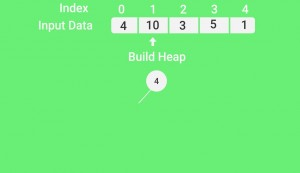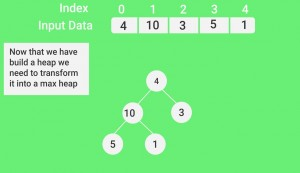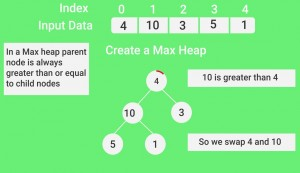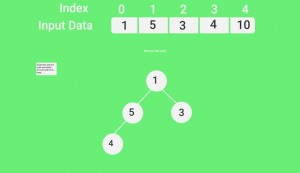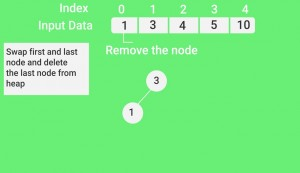Quiz on Heap Sort

Other Sorting Algorithms on GeeksforGeeks/GeeksQuiz:
QuickSort, Selection Sort, Bubble Sort, Insertion Sort, Merge Sort, Heap Sort, QuickSort, Radix Sort, Counting Sort, Bucket Sort, ShellSort, Comb Sort, Pigeonhole Sort

Coding practice for sorting.